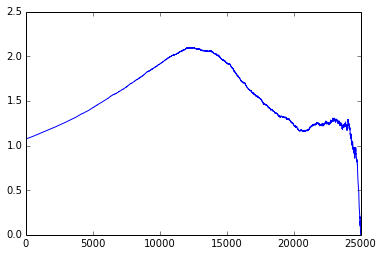# Starting kit for the Higgs boson machine learning challenge¶

This notebook contains a starting kit for the Higgs boson machine learning challenge. Download the training set (called training.csv) and the test set (test.csv), then execute cells in order.

In :
import random,string,math,csv
import numpy as np
import matplotlib.pyplot as plt


## Reading an formatting training data¶

In :
all = list(csv.reader(open("training.csv","rb"), delimiter=','))


Slicing off header row and id, weight, and label columns.

In :
xs = np.array([map(float, row[1:-2]) for row in all[1:]])
(numPoints,numFeatures) = xs.shape


Perturbing features to avoid ties. It's far from optimal but makes life easier in this simple example.

In :
xs = np.add(xs, np.random.normal(0.0, 0.0001, xs.shape))


Label selectors.

In :
sSelector = np.array([row[-1] == 's' for row in all[1:]])
bSelector = np.array([row[-1] == 'b' for row in all[1:]])


Weights and weight sums.

In :
weights = np.array([float(row[-2]) for row in all[1:]])
sumWeights = np.sum(weights)
sumSWeights = np.sum(weights[sSelector])
sumBWeights = np.sum(weights[bSelector])


## Training and validation cuts¶

We will train a classifier on a random training set for minimizing the weighted error with balanced weights, then we will maximize the AMS on the held out validation set.

In :
randomPermutation = random.sample(range(len(xs)), len(xs))
numPointsTrain = int(numPoints*0.9)
numPointsValidation = numPoints - numPointsTrain

xsTrain = xs[randomPermutation[:numPointsTrain]]
xsValidation = xs[randomPermutation[numPointsTrain:]]

sSelectorTrain = sSelector[randomPermutation[:numPointsTrain]]
bSelectorTrain = bSelector[randomPermutation[:numPointsTrain]]
sSelectorValidation = sSelector[randomPermutation[numPointsTrain:]]
bSelectorValidation = bSelector[randomPermutation[numPointsTrain:]]

weightsTrain = weights[randomPermutation[:numPointsTrain]]
weightsValidation = weights[randomPermutation[numPointsTrain:]]

sumWeightsTrain = np.sum(weightsTrain)
sumSWeightsTrain = np.sum(weightsTrain[sSelectorTrain])
sumBWeightsTrain = np.sum(weightsTrain[bSelectorTrain])

In :
xsTrainTranspose = xsTrain.transpose()


Making signal and background weights sum to $1/2$ each to emulate uniform priors $p(s)=p(b)=1/2$.

In :
weightsBalancedTrain = np.array([0.5 * weightsTrain[i]/sumSWeightsTrain
if sSelectorTrain[i]
else 0.5 * weightsTrain[i]/sumBWeightsTrain\
for i in range(numPointsTrain)])


## Training naive Bayes and defining the score function¶

Number of bins per dimension for binned naive Bayes.

In :
numBins = 10


logPs[fI,bI] will be the log probability of a data point x with binMaxs[bI - 1] < x[fI] <= binMaxs[bI] (with binMaxs[-1] = -$\infty$ by convention) being a signal under uniform priors $p(\text{s}) = p(\text{b}) = 1/2$.

In :
logPs = np.empty([numFeatures, numBins])
binMaxs = np.empty([numFeatures, numBins])
binIndexes = np.array(range(0, numPointsTrain+1, numPointsTrain/numBins))

In :
for fI in range(numFeatures):
# index permutation of sorted feature column
indexes = xsTrainTranspose[fI].argsort()

for bI in range(numBins):
# upper bin limits
binMaxs[fI, bI] = xsTrainTranspose[fI, indexes[binIndexes[bI+1]-1]]
# training indices of points in a bin
indexesInBin = indexes[binIndexes[bI]:binIndexes[bI+1]]
# sum of signal weights in bin
wS = np.sum(weightsBalancedTrain[indexesInBin]
[sSelectorTrain[indexesInBin]])
# sum of background weights in bin
wB = np.sum(weightsBalancedTrain[indexesInBin]
[bSelectorTrain[indexesInBin]])
# log probability of being a signal in the bin
logPs[fI, bI] = math.log(wS/(wS+wB))


The score function we will use to sort the test examples. For readability it is shifted so negative means likely background (under uniform prior) and positive means likely signal. x is an input vector.

In :
def score(x):
logP = 0
for fI in range(numFeatures):
bI = 0
# linear search for the bin index of the fIth feature
# of the signal
while bI < len(binMaxs[fI]) - 1 and x[fI] > binMaxs[fI, bI]:
bI += 1
logP += logPs[fI, bI] - math.log(0.5)
return logP


## Optimizing the AMS on the held out validation set¶

The Approximate Median Significance

s and b are the sum of signal and background weights, respectively, in the selection region.

In :
def AMS(s,b):
assert s >= 0
assert b >= 0
bReg = 10.
return math.sqrt(2 * ((s + b + bReg) *
math.log(1 + s / (b + bReg)) - s))


Computing the scores on the validation set

In :
validationScores = np.array([score(x) for x in xsValidation])


Sorting the indices in increasing order of the scores.

In :
tIIs = validationScores.argsort()


Weights have to be normalized to the same sum as in the full set.

In :
wFactor = 1.* numPoints / numPointsValidation


Initializing $s$ and $b$ to the full sum of weights, we start by having all points in the selectiom region.

In :
s = np.sum(weightsValidation[sSelectorValidation])
b = np.sum(weightsValidation[bSelectorValidation])


amss will contain AMSs after each point moved out of the selection region in the sorted validation set.

In :
amss = np.empty([len(tIIs)])


amsMax will contain the best validation AMS, and threshold will be the smallest score among the selected points.

In :
amsMax = 0
threshold = 0.0


We will do len(tIIs) iterations, which means that amss[-1] is the AMS when only the point with the highest score is selected.

In :
for tI in range(len(tIIs)):
# don't forget to renormalize the weights to the same sum
# as in the complete training set
amss[tI] = AMS(max(0,s * wFactor),max(0,b * wFactor))
if amss[tI] > amsMax:
amsMax = amss[tI]
threshold = validationScores[tIIs[tI]]
#print tI,threshold
if sSelectorValidation[tIIs[tI]]:
s -= weightsValidation[tIIs[tI]]
else:
b -= weightsValidation[tIIs[tI]]

In :
amsMax

Out:
2.0981127820868344

In :
threshold

Out:
-0.27902225726337126

In :
plt.plot(amss)

Out:
[<matplotlib.lines.Line2D at 0x123603490>]## Computing the permutation on the test set¶

Reading the test file, slicing off the header row and the id column, and converting the data into float.

In :
test = list(csv.reader(open("test.csv", "rb"),delimiter=','))
xsTest = np.array([map(float, row[1:]) for row in test[1:]])

In :
testIds = np.array([int(row) for row in test[1:]])


Computing the scores.

In :
testScores = np.array([score(x) for x in xsTest])


Computing the rank order.

In :
testInversePermutation = testScores.argsort()

In :
testPermutation = list(testInversePermutation)
for tI,tII in zip(range(len(testInversePermutation)),
testInversePermutation):
testPermutation[tII] = tI


Computing the submission file with columns EventId, RankOrder, and Class.

In :
submission = np.array([[str(testIds[tI]),str(testPermutation[tI]+1),
's' if testScores[tI] >= threshold else 'b']
for tI in range(len(testIds))])

In :
submission = np.append([['EventId','RankOrder','Class']],
submission, axis=0)


Saving the file that can be submitted to Kaggle.

In :
np.savetxt("submission.csv",submission,fmt='%s',delimiter=',')